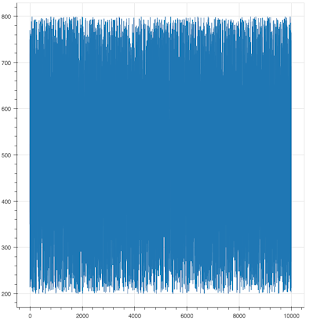# Remove padding to on inside of a chart

Is there any way to remove the padding that surrounds the lines/glyphs inside of a chart?

In the example below I would like to remove the left and right space/padding so that x=0 is inline with the y axis and x=10000 is inline with the right-hand edge of the plot.

Many thanks,

Alex

``````from bokeh.plotting import figure, output_file, show
import numpy as np

output_file("mwe.html")
p = figure()
p.line(np.arange(0, 10000, 1), np.random.randint(200, 800, 10000), line_width=1)
show(p)

``````Hi,

Yes, see

Thanks,

Bryan

···

On Mar 5, 2018, at 03:56, [email protected] wrote:

Is there any way to remove the padding that surrounds the lines/glyphs inside of a chart?

In the example below I would like to remove the left and right space/padding so that x=0 is inline with the y axis and x=10000 is inline with the right-hand edge of the plot.

Many thanks,

Alex

from bokeh.plotting import figure, output_file, show
import numpy as np

output_file("mwe.html")
p = figure()
p.line(np.arange(0, 10000, 1), np.random.randint(200, 800, 10000), line_width=1)
show(p)

--
You received this message because you are subscribed to the Google Groups "Bokeh Discussion - Public" group.
To unsubscribe from this group and stop receiving emails from it, send an email to [email protected].
To post to this group, send email to [email protected].
To view this discussion on the web visit https://groups.google.com/a/continuum.io/d/msgid/bokeh/c4493e2c-fbc4-446d-a3a2-95ff3ffd2a7d%40continuum.io.